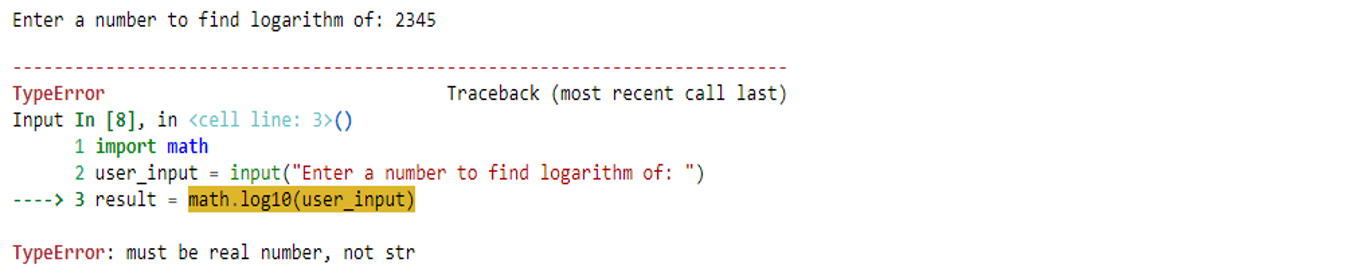Tutorial

## Python must be real number not str

##### Python Tutor

When doing math-related coding with Python, we might run into the error `TypeError: must be real number, not str`. This error can be puzzling to those new to the language, but in this article, we will demystify the error, diving into why the error might occur, and how to solve it.

## Understanding `TypeError: must be real number, not str`

The error `TypeError: must be real number not str`will occur whenever a mathematical operation is carried out on a string (rather than a float or integer). There are several scenarios in which we can encounter the error, and we will examine some of them in the subsections that follow.

### User Input

On common case that we can encounter the error is when requesting user input. The `input` function returns the user entry as a string, but we make use of the input as it is where a `float` or `int` was expected. For example:

``````import math
response = input("Enter a number to find logarithm of: ")
result = math.log10(response)
print(result)``````

Our code example wants to find the logarithm (base 10) of a number entered by the user. When our code is executed, we get the following;The program requests user input, and then prints out an error message `TypeError: must be real number, not str` since we are trying to find the logarithm of a string value.

To remove the error message, we have to parse the user input string into a `float` before making use of it. We can do this using the `float()` function. The next example has the same code but with the corrections effected to remove the error message;

``````import math
response = float(input("Enter a number to find logarithm of: "))
result = math.log10(response)
print(result)``````

This version of our code works fine, as we do not have any error message displayed on the output. The output we get this time looks like this;In the code, we passed the return value of the `input()`function as an argument of `float()`. The `math.log10`function now has the correct data type to use in its calculations. Alternatively, we could do the following;

``````import math
response = input("Enter a number to find logarithm of: ")
result = math.log10(float(response))
print(result)``````

The above code also works well. The key is to carry out the conversion before the function `math.log10` makes use of the `str` value from the user input.

### Data Processing

In the second scenario, we will try to read values from a `data.csv`file, find the sum of each row and write the results to a `results.csv`file. The contents of the `data.csv`file is as follows;Our `data.csv` file contains three rows of data; each row has two columns of `float`data (as `str`since everything in the CSV file is a string). The following code tries to read the `data.csv`, sum of each column, then write the results to an output file.

However, our code will not run successfully.

``````import math
import csv

# Open the input and output CSV files
with open('data.csv', 'r') as rcsv, open('result.csv', 'w', newline='') as wcsv:
writer = csv.writer(wcsv)

value1 = row
value2 = row
total = math.fsum([value1, value2])

# Write the row with sum to the output file
writer.writerow([value1, value2, total])

print(f"Results successfully written to 'results.csv'.")``````

Can you find out why our code will display an error message?

It is because while making use of `math.fsum`, we did not convert the values from the row to float. Instead, we passed a list of `str`objects into `math.fsum`. See the output below for the error message.What we simply need to do is to convert each of `value1` and `value2`to `float`type before passing them to `math.fsum`. The corrected code below shows (in bold) the corrections made to our previous code;

``````import math
import csv

# Open the input and output CSV files
with open('data.csv', 'r') as rcsv, open('result.csv', 'w', newline='') as wcsv:
writer = csv.writer(wcsv)

value1 = row
value2 = row
total = math.fsum([float(value1), float(value2)])

# Write the row with sum to the output file
writer.writerow([value1, value2, total])

print(f"Results successfully written to 'results.csv'.")``````

This time, we do not get an error message because `math.fsum` is getting `float`arguments, rather than `str`. The `print` function is also successfully executed, as shown below.If we take a peek into our output `.csv` file (`results.csv`) we see that our code produced the expected output successfully.The `results.csv` file has three columns now since we added an extra `sum` column to hold the sum of entries in the previous columns.

The error `TypeError: must be real number, not str` is a common pitfall for Python programmers. however, as we have seen in the above code examples, it can be easily resolved by converting the `str`value into an appropriate numeric type. This will allow your code to behave as expected.

If you liked this tutorial, please you are welcome to check out our Python free tutorial that will certainly help you solve common coding problems in Python. Or if you need a dedicated tutor for Python then contact us.